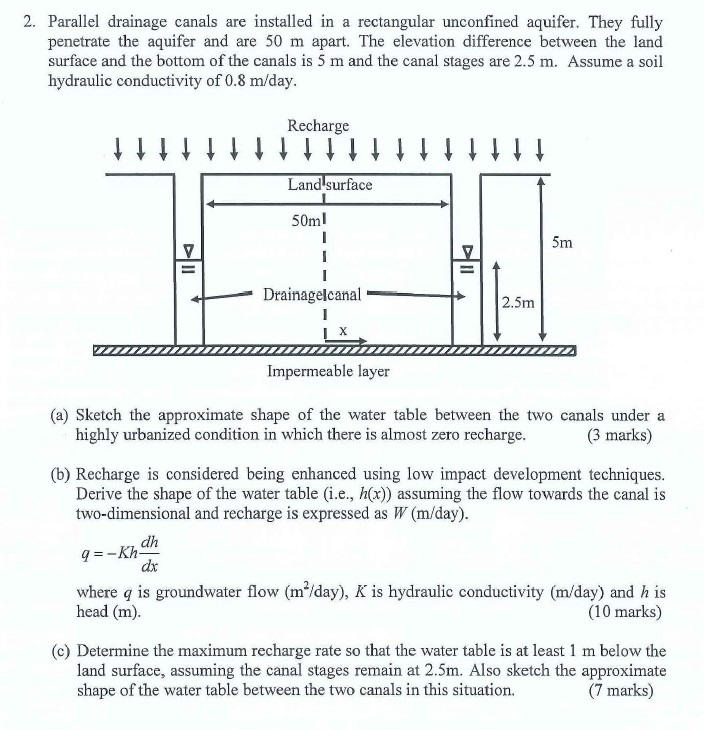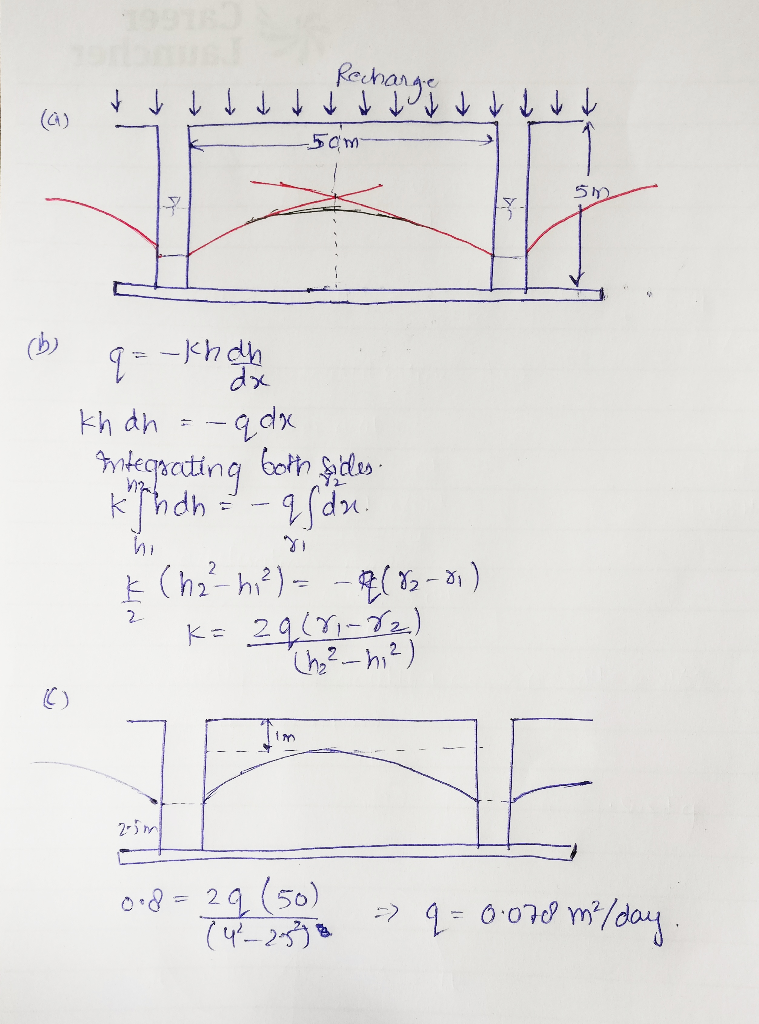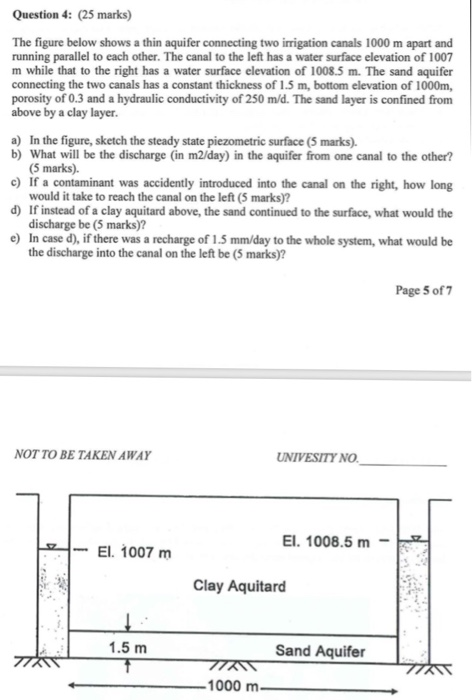# 2. Parallel drainage canals are installed in a rectangular unconfined aquifer. They fully penetrate the aquifer and are 50 m apart. The elevation difference between the land surface and the bottom of...2. Parallel drainage canals are installed in a rectangular unconfined aquifer. They fully penetrate the aquifer and are 50 m apart. The elevation difference between the land surface and the bottom of the canals is 5 m and the canal stages are 2.5 m. Assume a soil hydraulic conductivity of 0.8 m/day Recharge Land surface 50ml Drainagelcanal 2.5m Impermeable layer (a) Sketch the approximate shape of the water table between the two canals under a highly urbanized condition in which there is almost zero recharge (3 marks) (b) Recharge is considered being enhanced using low impact development techniques Derive the shape of the water table (i.e., h(x)) assuming the flow towards the canal is two-dimensional and recharge is expressed as W (m/day) dh dx where q is groundwater flow (m2/day), K is hydraulic conductivity (m/day) and h is head (m) (10 marks) (c) Determine the maximum recharge rate so that the water table is at least 1 m below the land surface, assuming the canal stages remain at 2.5m. Also sketch the approximate shape of the water table between the two canals in this situation. (7 marks)#### Post as a guestQuestion 4: (25 marks) The figure below shows a thin aquifer connecting two irrigation canals 1000 m apart and running parallel to each other. The canal to the left has a water surface elevation of 1007 m while that to the right has a water surface elevation of 1008.5 m. The sand aquifer connecting the two canals has a constant thickness of 1.5 m, bottom elevation of 1000m, porosity of 0.3 and a hydraulic conductivity of 250 m/d. The sand...